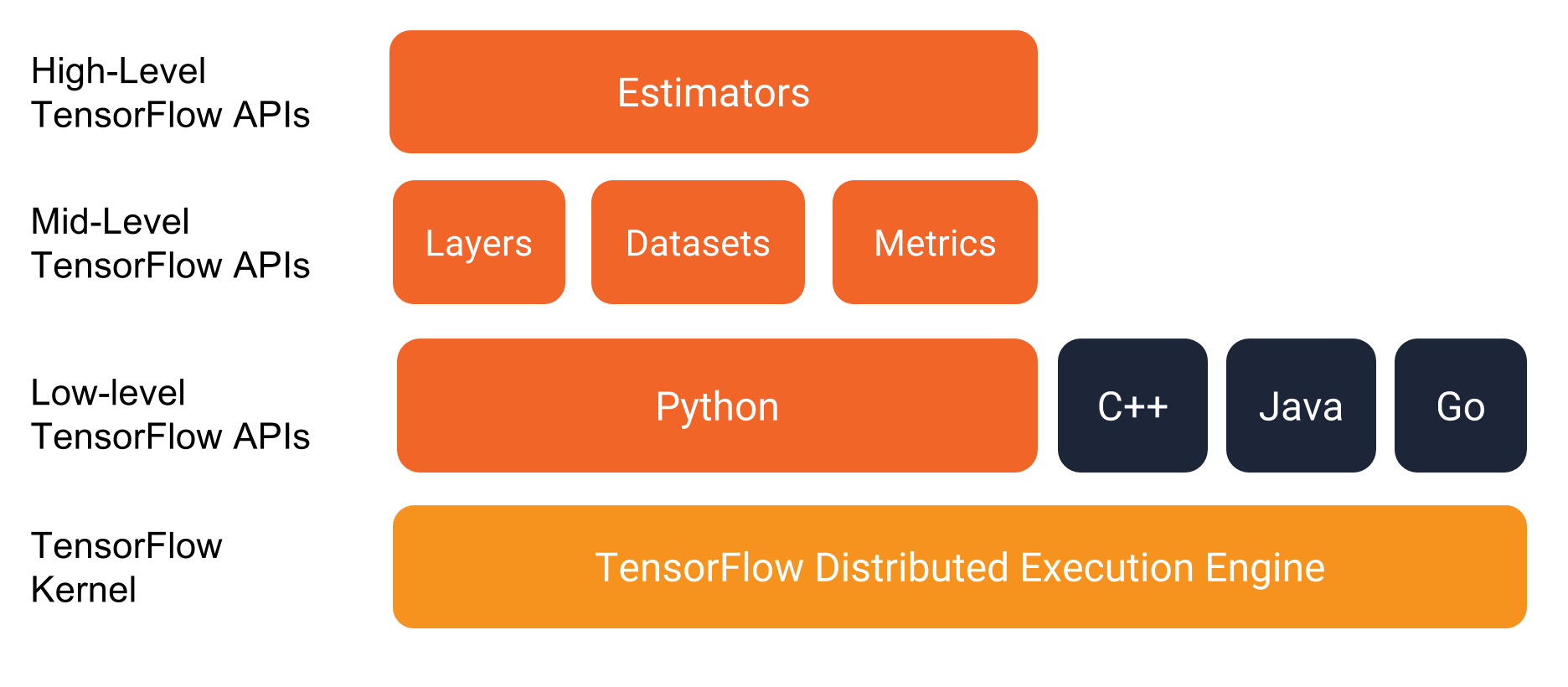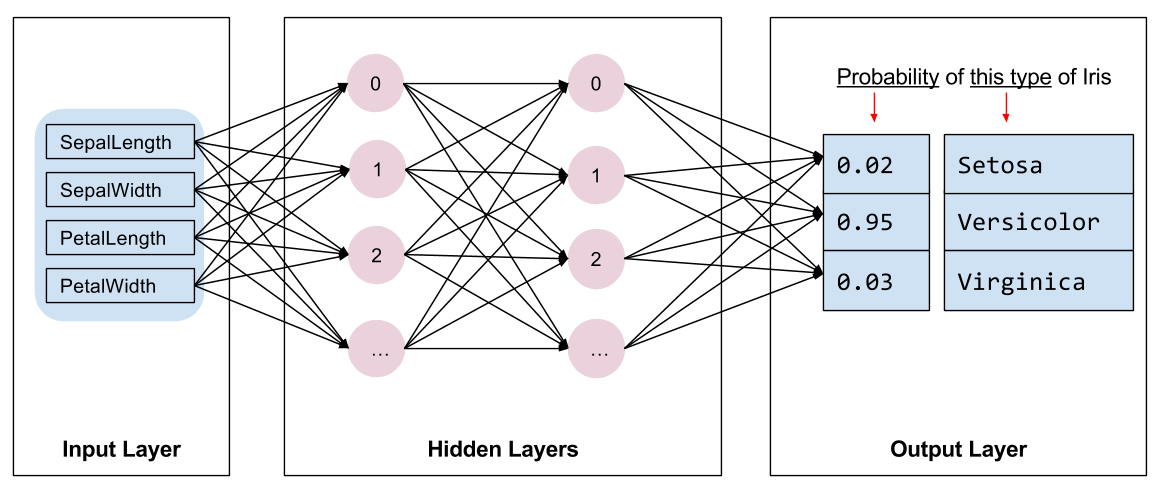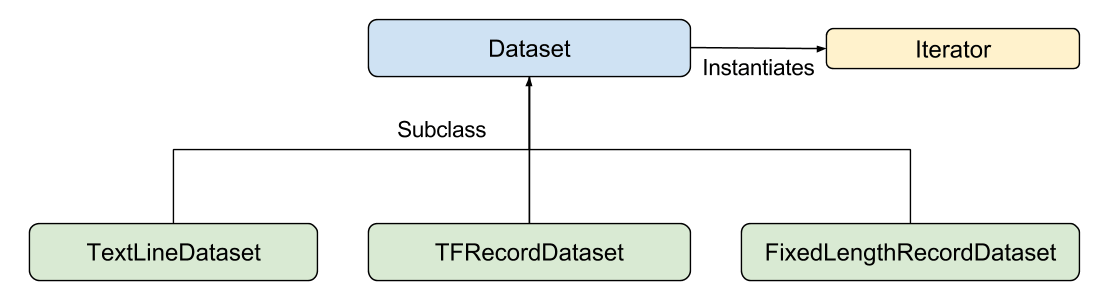# W3cubDocs

/TensorFlow Guide

This document introduces the TensorFlow programming environment and shows you how to solve the Iris classification problem in TensorFlow.

## Prerequisites

Prior to using the sample code in this document, you'll need to do the following:

• Install TensorFlow.
• If you installed TensorFlow with virtualenv or Anaconda, activate your TensorFlow environment.
• Install or upgrade pandas by issuing the following command:

```pip install pandas
```

## Getting the sample code

Take the following steps to get the sample code we'll be going through:

1. Clone the TensorFlow Models repository from GitHub by entering the following command:

```git clone https://github.com/tensorflow/models
```
2. Change directory within that branch to the location containing the examples used in this document:

```cd models/samples/core/get_started/
```

The program described in this document is `premade_estimator.py`. This program uses `iris_data.py` to fetch its training data.

### Running the program

You run TensorFlow programs as you would run any Python program. For example:

```python premade_estimator.py
```

The program should output training logs followed by some predictions against the test set. For example, the first line in the following output shows that the model thinks there is a 99.6% chance that the first example in the test set is a Setosa. Since the test set expected Setosa, this appears to be a good prediction.

```...
Prediction is "Setosa" (99.6%), expected "Setosa"

Prediction is "Versicolor" (99.8%), expected "Versicolor"

Prediction is "Virginica" (97.9%), expected "Virginica"
```

If the program generates errors instead of answers, ask yourself the following questions:

• Did you install TensorFlow properly?
• Are you using the correct version of TensorFlow?
• Did you activate the environment you installed TensorFlow in? (This is only relevant in certain installation mechanisms.)

## The programming stack

Before getting into the details of the program itself, let's investigate the programming environment. As the following illustration shows, TensorFlow provides a programming stack consisting of multiple API layers:We strongly recommend writing TensorFlow programs with the following APIs:

• Estimators, which represent a complete model. The Estimator API provides methods to train the model, to judge the model's accuracy, and to generate predictions.
• Datasets, which build a data input pipeline. The Dataset API has methods to load and manipulate data, and feed it into your model. The Dataset API meshes well with the Estimators API.

## Classifying irises: an overview

The sample program in this document builds and tests a model that classifies Iris flowers into three different species based on the size of their sepals and petals.From left to right, Iris setosa (by Radomil, CC BY-SA 3.0), Iris versicolor (by Dlanglois, CC BY-SA 3.0), and Iris virginica (by Frank Mayfield, CC BY-SA 2.0).

### The data set

The Iris data set contains four features and one label. The four features identify the following botanical characteristics of individual Iris flowers:

• sepal length
• sepal width
• petal length
• petal width

Our model will represent these features as `float32` numerical data.

The label identifies the Iris species, which must be one of the following:

• Iris setosa (0)
• Iris versicolor (1)
• Iris virginica (2)

Our model will represent the label as `int32` categorical data.

The following table shows three examples in the data set:

sepal length sepal width petal length petal width species (label)
5.1 3.3 1.7 0.5 0 (Setosa)
5.0 2.3 3.3 1.0 1 (versicolor)
6.4 2.8 5.6 2.2 2 (virginica)

### The algorithm

The program trains a Deep Neural Network classifier model having the following topology:

• 2 hidden layers.
• Each hidden layer contains 10 nodes.

The following figure illustrates the features, hidden layers, and predictions (not all of the nodes in the hidden layers are shown):### Inference

Running the trained model on an unlabeled example yields three predictions, namely, the likelihood that this flower is the given Iris species. The sum of those output predictions will be 1.0. For example, the prediction on an unlabeled example might be something like the following:

• 0.03 for Iris Setosa
• 0.95 for Iris Versicolor
• 0.02 for Iris Virginica

The preceding prediction indicates a 95% probability that the given unlabeled example is an Iris Versicolor.

## Overview of programming with Estimators

An Estimator is TensorFlow's high-level representation of a complete model. It handles the details of initialization, logging, saving and restoring, and many other features so you can concentrate on your model. For more details see Estimators.

An Estimator is any class derived from `tf.estimator.Estimator`. TensorFlow provides a collection of pre-made Estimators (for example, `LinearRegressor`) to implement common ML algorithms. Beyond those, you may write your own custom Estimators. We recommend using pre-made Estimators when just getting started with TensorFlow. After gaining expertise with the pre-made Estimators, we recommend optimizing your model by creating your own custom Estimators.

To write a TensorFlow program based on pre-made Estimators, you must perform the following tasks:

• Create one or more input functions.
• Define the model's feature columns.
• Instantiate an Estimator, specifying the feature columns and various hyperparameters.
• Call one or more methods on the Estimator object, passing the appropriate input function as the source of the data.

Let's see how those tasks are implemented for Iris classification.

## Create input functions

You must create input functions to supply data for training, evaluating, and prediction.

An input function is a function that returns a `tf.data.Dataset` object which outputs the following two-element tuple:

• `features` - A Python dictionary in which:
• Each key is the name of a feature.
• Each value is an array containing all of that feature's values.
• `label` - An array containing the values of the label for every example.

Just to demonstrate the format of the input function, here's a simple implementation:

```def input_evaluation_set():
features = {'SepalLength': np.array([6.4, 5.0]),
'SepalWidth':  np.array([2.8, 2.3]),
'PetalLength': np.array([5.6, 3.3]),
'PetalWidth':  np.array([2.2, 1.0])}
labels = np.array([2, 1])
return features, labels
```

Your input function may generate the `features` dictionary and `label` list any way you like. However, we recommend using TensorFlow's Dataset API, which can parse all sorts of data. At a high level, the Dataset API consists of the following classes:Where the individual members are:

• `Dataset` - Base class containing methods to create and transform datasets. Also allows you to initialize a dataset from data in memory, or from a Python generator.
• `TextLineDataset` - Reads lines from text files.
• `TFRecordDataset` - Reads records from TFRecord files.
• `FixedLengthRecordDataset` - Reads fixed size records from binary files.
• `Iterator` - Provides a way to access one data set element at a time.

The Dataset API can handle a lot of common cases for you. For example, using the Dataset API, you can easily read in records from a large collection of files in parallel and join them into a single stream.

To keep things simple in this example we are going to load the data with pandas, and build our input pipeline from this in-memory data.

Here is the input function used for training in this program, which is available in `iris_data.py`:

```def train_input_fn(features, labels, batch_size):
"""An input function for training"""
# Convert the inputs to a Dataset.
dataset = tf.data.Dataset.from_tensor_slices((dict(features), labels))

# Shuffle, repeat, and batch the examples.
return dataset.shuffle(1000).repeat().batch(batch_size)
```

## Define the feature columns

A feature column is an object describing how the model should use raw input data from the features dictionary. When you build an Estimator model, you pass it a list of feature columns that describes each of the features you want the model to use. The `tf.feature_column` module provides many options for representing data to the model.

For Iris, the 4 raw features are numeric values, so we'll build a list of feature columns to tell the Estimator model to represent each of the four features as 32-bit floating-point values. Therefore, the code to create the feature column is:

```# Feature columns describe how to use the input.
my_feature_columns = []
for key in train_x.keys():
my_feature_columns.append(tf.feature_column.numeric_column(key=key))
```

Feature columns can be far more sophisticated than those we're showing here. We detail feature columns later on in our Getting Started guide.

Now that we have the description of how we want the model to represent the raw features, we can build the estimator.

## Instantiate an estimator

The Iris problem is a classic classification problem. Fortunately, TensorFlow provides several pre-made classifier Estimators, including:

For the Iris problem, `tf.estimator.DNNClassifier` seems like the best choice. Here's how we instantiated this Estimator:

```# Build a DNN with 2 hidden layers and 10 nodes in each hidden layer.
classifier = tf.estimator.DNNClassifier(
feature_columns=my_feature_columns,
# Two hidden layers of 10 nodes each.
hidden_units=[10, 10],
# The model must choose between 3 classes.
n_classes=3)
```

## Train, Evaluate, and Predict

Now that we have an Estimator object, we can call methods to do the following:

• Train the model.
• Evaluate the trained model.
• Use the trained model to make predictions.

### Train the model

Train the model by calling the Estimator's `train` method as follows:

```# Train the Model.
classifier.train(
input_fn=lambda:iris_data.train_input_fn(train_x, train_y, args.batch_size),
steps=args.train_steps)
```

Here we wrap up our `input_fn` call in a `lambda` to capture the arguments while providing an input function that takes no arguments, as expected by the Estimator. The `steps` argument tells the method to stop training after a number of training steps.

### Evaluate the trained model

Now that the model has been trained, we can get some statistics on its performance. The following code block evaluates the accuracy of the trained model on the test data:

```# Evaluate the model.
eval_result = classifier.evaluate(
input_fn=lambda:iris_data.eval_input_fn(test_x, test_y, args.batch_size))

print('\nTest set accuracy: {accuracy:0.3f}\n'.format(**eval_result))
```

Unlike our call to the `train` method, we did not pass the `steps` argument to evaluate. Our `eval_input_fn` only yields a single epoch of data.

Running this code yields the following output (or something similar):

```Test set accuracy: 0.967
```

### Making predictions (inferring) from the trained model

We now have a trained model that produces good evaluation results. We can now use the trained model to predict the species of an Iris flower based on some unlabeled measurements. As with training and evaluation, we make predictions using a single function call:

```# Generate predictions from the model
expected = ['Setosa', 'Versicolor', 'Virginica']
predict_x = {
'SepalLength': [5.1, 5.9, 6.9],
'SepalWidth': [3.3, 3.0, 3.1],
'PetalLength': [1.7, 4.2, 5.4],
'PetalWidth': [0.5, 1.5, 2.1],
}

predictions = classifier.predict(
input_fn=lambda:iris_data.eval_input_fn(predict_x,
batch_size=args.batch_size))
```

The `predict` method returns a Python iterable, yielding a dictionary of prediction results for each example. The following code prints a few predictions and their probabilities:

```template = ('\nPrediction is "{}" ({:.1f}%), expected "{}"')

for pred_dict, expec in zip(predictions, expected):
class_id = pred_dict['class_ids']
probability = pred_dict['probabilities'][class_id]

print(template.format(iris_data.SPECIES[class_id],
100 * probability, expec))
```

Running the preceding code yields the following output:

```...
Prediction is "Setosa" (99.6%), expected "Setosa"

Prediction is "Versicolor" (99.8%), expected "Versicolor"

Prediction is "Virginica" (97.9%), expected "Virginica"
```

## Summary

Pre-made Estimators are an effective way to quickly create standard models.

Now that you've gotten started writing TensorFlow programs, consider the following material: